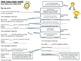Subject
Resource Type
File Type

PDF

(2 MB)
Standards
• Product Description
• StandardsNEW
TIME CHALLENGE

“Like the big kids, but better!”

TIME CHALLENGE (2 SETS)
Let your students experience solving math problems under the clock. I included 6 addition worksheets and 6 subtraction worksheets. Give the students a set time to finish each worksheet. Work with them at setting goals for improving their scores. Special TIME CHALLENGE sheets for recording each student’s scores and goals included.

Make it fun!
They can be awarded a gold ribbon or trophy (clip art attached) for finishing all six sheets. For a perfect score perhaps give out an extra reward. I attached some bookmarks. They are very colorful, but even nicer if laminated!

TIME CHALLENGE SETS
6 Time Challenge Worksheets (Solve the Problems. Find the Numbers)/Answer Keys Provided
The worksheets are designed with a racing motorcycle and each sheet shows the motorcycle moving closer to the finish line! The last sheet shows the motorcycle reaching the finish line. Every possible combination of numbers included on the sheets, with the sum always within 0 – 10.

SUBTRACTION
6 Worksheets (Solve the Problems. Find the Numbers)/Answer Keys Provided
The worksheets are designed with a racing car and each sheet shows the car moving closer to the finish line! The last sheet shows the car reaching the finish line. Every possible combination of numbers included on the sheets, with the sum always within 0 – 10.

A fun and helpful way to meet the following Common Core Standards:
CCSS.Math.Content.1.OA.B.3
CCSS.Math.Content.1.OA.B.4
CCSS.Math.Content.1.OA.C.5
CCSS.Math.Content.1.OA.C.6
CCSS.Math.Content.1.OA.D.7
CCSS.Math.Content.1.OA.D.8

All the 1st Grade CCS products with my colorful RACE CAR THEME:

Other helpful products with my colorful RACE CARE THEME:
Hundreds Chart with Worksheets
FLASHCARDS: Numbers 0 to 100 - Matching Hundreds Chart
FLASHCARDS: Numbers 0 to 100 - Double Sided with Counting Grid

Determine the unknown whole number in an addition or subtraction equation relating three whole numbers. For example, determine the unknown number that makes the equation true in each of the equations 8 + ? = 11, 5 = ▯ - 3, 6 + 6 = ▯.
Add and subtract within 20, demonstrating fluency for addition and subtraction within 10. Use strategies such as counting on; making ten (e.g., 8 + 6 = 8 + 2 + 4 = 10 + 4 = 14); decomposing a number leading to a ten (e.g., 13 - 4 = 13 - 3 - 1 = 10 - 1 = 9); using the relationship between addition and subtraction (e.g., knowing that 8 + 4 = 12, one knows 12 - 8 = 4); and creating equivalent but easier or known sums (e.g., adding 6 + 7 by creating the known equivalent 6 + 6 + 1 = 12 + 1 = 13).
Relate counting to addition and subtraction (e.g., by counting on 2 to add 2).
Understand subtraction as an unknown-addend problem. For example, subtract 10 – 8 by finding the number that makes 10 when added to 8.
Apply properties of operations as strategies to add and subtract. If 8 + 3 = 11 is known, then 3 + 8 = 11 is also known. (Commutative property of addition.) To add 2 + 6 + 4, the second two numbers can be added to make a ten, so 2 + 6 + 4 = 2 + 10 = 12. (Associative property of addition.)
Total Pages
N/A
N/A
Teaching Duration
N/A
Report this Resource to TpT
Reported resources will be reviewed by our team. Report this resource to let us know if this resource violates TpT’s content guidelines.